# An Overview of MOD Function | Microsoft Excel

The MOD function is one of the math and trigonometry functions in Microsoft Excel. It can be used as a standalone function as well as in collaboration with the other functions. This article will give you an overview of the MOD function in Excel.

### Objectives

The MOD function calculates the remainder when a numerical value (dividend) is divided by another one (divisor).

### Syntax

`=MOD(number, divisor)`

### Arguments

• number: Compulsory. It refers to the dividend i.e. the number to divide.
• divisor: Compulsory. The number by which you want to divide the dividend.

### Output

A numerical value i.e. the remainder.

## Examples of MOD Function

Suppose, you have the number 39 in cell A2. Then you have a divisor 5 in cell B2. Let’s say you want to calculate the remainder if you divide 39 by 5. Well, you can use the MOD function to get the remainder quite easily. For that, use the formula:

`=MOD(A2,B2)`

The output will be 4.

Here are some examples of the usage of the MOD function.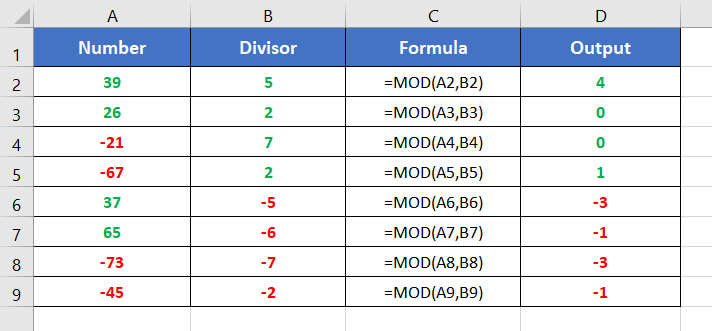## Usage Guide of MOD Function

Step_1: Start with inserting an equal sign (=) in a blank cell.

Step_2: Then type MOD.

Step_3: Type open parenthesis “(“.

As you type the open parenthesis, Excel shows the syntax of the MOD function.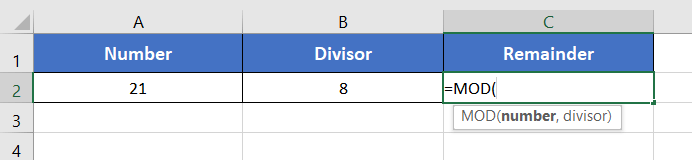Step_4: Insert a number first.

You can directly insert a number or a cell address that contains a number.

Here, I’m inserting A2 which has the number 21.

Step_5: Then insert a comma (,).

After inserting the comma, Excel will ask you for the second argument which is the divisor.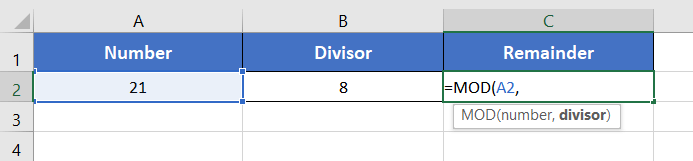Step_6: Now insert the divisor.

You can insert the cell address of the divisor or directly insert a number as a divisor.

Here, the divisor is in cell B2. So, I’m inserting B2 inside the function.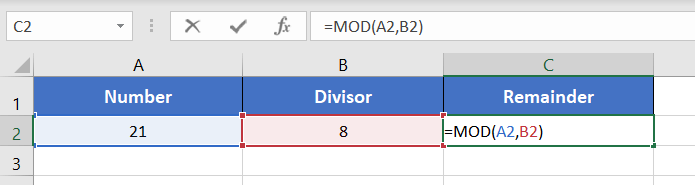Final Result

The MOD function returns 5. Because, when you divide 21 by 8, the remainder is 5. Thus, the output is 5.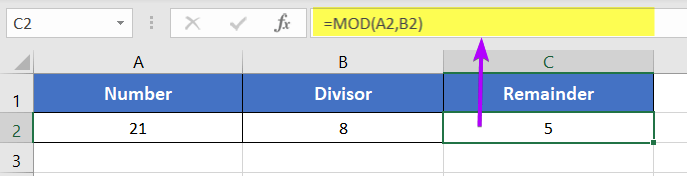### Availability

 Excel for Microsoft 365 Excel 2016 Excel for Microsoft 365 for Mac Excel 2016 for Mac Excel for the web Excel 2013 Excel 2021 Excel 2010 Excel 2021 for Mac Excel 2007 Excel 2019 Excel for Mac 2011 Excel 2019 for Mac Excel Starter 2010

### Remarks

• The MOD function shows #VALUE! error in the case of non-numeric values.
• The sign of the remainder is the same as the sign of the divisor. This means for the positive divisor, the remainder becomes positive. For a negative divisor, the remainder becomes negative.

## Conclusion

The MOD function is one of the math and trigonometry functions in Excel. If you have any questions regarding the MOD function, please comment below. Thanks!